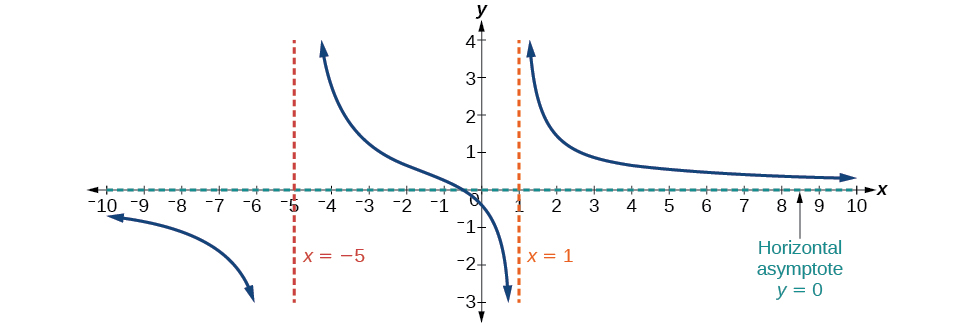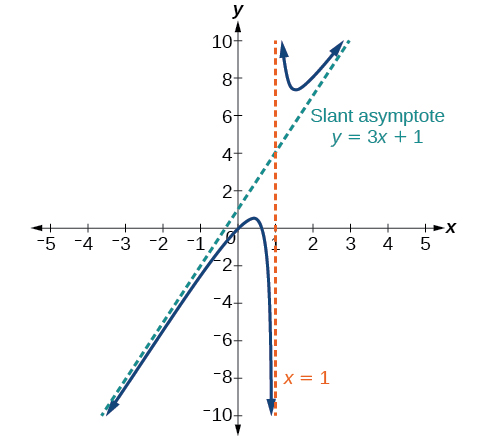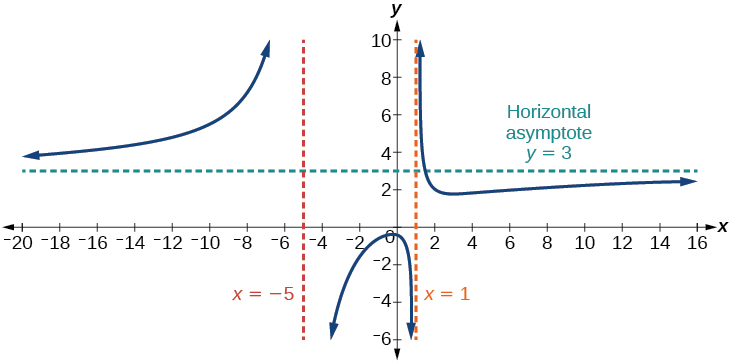# 5.6 Rational functions  (Page 5/16)

 Page 5 / 16

Find the vertical asymptotes and removable discontinuities of the graph of $\text{\hspace{0.17em}}f\left(x\right)=\frac{{x}^{2}-25}{{x}^{3}-6{x}^{2}+5x}.$

Removable discontinuity at $\text{\hspace{0.17em}}x=5.\text{\hspace{0.17em}}$ Vertical asymptotes:

## Identifying horizontal asymptotes of rational functions

While vertical asymptotes describe the behavior of a graph as the output gets very large or very small, horizontal asymptotes help describe the behavior of a graph as the input gets very large or very small. Recall that a polynomial’s end behavior will mirror that of the leading term. Likewise, a rational function’s end behavior will mirror that of the ratio of the function that is the ratio of the leading terms.

There are three distinct outcomes when checking for horizontal asymptotes:

Case 1: If the degree of the denominator>degree of the numerator, there is a horizontal asymptote    at $\text{\hspace{0.17em}}y=0.$

In this case, the end behavior is $\text{\hspace{0.17em}}f\left(x\right)\approx \frac{4x}{{x}^{2}}=\frac{4}{x}.\text{\hspace{0.17em}}$ This tells us that, as the inputs increase or decrease without bound, this function will behave similarly to the function $\text{\hspace{0.17em}}g\left(x\right)=\frac{4}{x},\text{\hspace{0.17em}}$ and the outputs will approach zero, resulting in a horizontal asymptote at $\text{\hspace{0.17em}}y=0.\text{\hspace{0.17em}}$ See [link] . Note that this graph crosses the horizontal asymptote.Horizontal asymptote   y = 0   when   f ( x ) = p ( x ) q ( x ) ,   q ( x ) ≠ 0   where degree of   p < degree of  q .

Case 2: If the degree of the denominator<degree of the numerator by one, we get a slant asymptote.

In this case, the end behavior is $\text{\hspace{0.17em}}f\left(x\right)\approx \frac{3{x}^{2}}{x}=3x.\text{\hspace{0.17em}}$ This tells us that as the inputs increase or decrease without bound, this function will behave similarly to the function $\text{\hspace{0.17em}}g\left(x\right)=3x.\text{\hspace{0.17em}}$ As the inputs grow large, the outputs will grow and not level off, so this graph has no horizontal asymptote. However, the graph of $\text{\hspace{0.17em}}g\left(x\right)=3x\text{\hspace{0.17em}}$ looks like a diagonal line, and since $\text{\hspace{0.17em}}f\text{\hspace{0.17em}}$ will behave similarly to $\text{\hspace{0.17em}}g,\text{\hspace{0.17em}}$ it will approach a line close to $\text{\hspace{0.17em}}y=3x.\text{\hspace{0.17em}}$ This line is a slant asymptote.

To find the equation of the slant asymptote, divide $\text{\hspace{0.17em}}\frac{3{x}^{2}-2x+1}{x-1}.\text{\hspace{0.17em}}$ The quotient is $\text{\hspace{0.17em}}3x+1,\text{\hspace{0.17em}}$ and the remainder is 2. The slant asymptote is the graph of the line $\text{\hspace{0.17em}}g\left(x\right)=3x+1.\text{\hspace{0.17em}}$ See [link] .Slant asymptote when   f ( x ) = p ( x ) q ( x ) ,   q ( x ) ≠ 0   where degree of   p > degree of  q   by   1 .

Case 3: If the degree of the denominator = degree of the numerator, there is a horizontal asymptote at $\text{\hspace{0.17em}}y=\frac{{a}_{n}}{{b}_{n}},\text{\hspace{0.17em}}$ where $\text{\hspace{0.17em}}{a}_{n}\text{\hspace{0.17em}}$ and $\text{\hspace{0.17em}}{b}_{n}\text{\hspace{0.17em}}$ are the leading coefficients of $\text{\hspace{0.17em}}p\left(x\right)\text{\hspace{0.17em}}$ and $\text{\hspace{0.17em}}q\left(x\right)\text{\hspace{0.17em}}$ for $\text{\hspace{0.17em}}f\left(x\right)=\frac{p\left(x\right)}{q\left(x\right)},q\left(x\right)\ne 0.$

In this case, the end behavior is $\text{\hspace{0.17em}}f\left(x\right)\approx \frac{3{x}^{2}}{{x}^{2}}=3.\text{\hspace{0.17em}}$ This tells us that as the inputs grow large, this function will behave like the function $\text{\hspace{0.17em}}g\left(x\right)=3,\text{\hspace{0.17em}}$ which is a horizontal line. As $\text{\hspace{0.17em}}x\to ±\infty ,f\left(x\right)\to 3,\text{\hspace{0.17em}}$ resulting in a horizontal asymptote at $\text{\hspace{0.17em}}y=3.\text{\hspace{0.17em}}$ See [link] . Note that this graph crosses the horizontal asymptote.Horizontal asymptote when   f ( x ) = p ( x ) q ( x ) ,   q ( x ) ≠ 0   where degree of  p = degree of  q .

Notice that, while the graph of a rational function will never cross a vertical asymptote    , the graph may or may not cross a horizontal or slant asymptote. Also, although the graph of a rational function may have many vertical asymptotes, the graph will have at most one horizontal (or slant) asymptote.

#### Questions & Answers

write down the polynomial function with root 1/3,2,-3 with solution
if A and B are subspaces of V prove that (A+B)/B=A/(A-B)
write down the value of each of the following in surd form a)cos(-65°) b)sin(-180°)c)tan(225°)d)tan(135°)
Prove that (sinA/1-cosA - 1-cosA/sinA) (cosA/1-sinA - 1-sinA/cosA) = 4
what is the answer to dividing negative index
In a triangle ABC prove that. (b+c)cosA+(c+a)cosB+(a+b)cisC=a+b+c.
give me the waec 2019 questions
the polar co-ordinate of the point (-1, -1)
prove the identites sin x ( 1+ tan x )+ cos x ( 1+ cot x )= sec x + cosec x
tanh`(x-iy) =A+iB, find A and B
B=Ai-itan(hx-hiy)
Rukmini
what is the addition of 101011 with 101010
If those numbers are binary, it's 1010101. If they are base 10, it's 202021.
Jack
extra power 4 minus 5 x cube + 7 x square minus 5 x + 1 equal to zero
the gradient function of a curve is 2x+4 and the curve passes through point (1,4) find the equation of the curve
1+cos²A/cos²A=2cosec²A-1
test for convergence the series 1+x/2+2!/9x3ByByByByBy